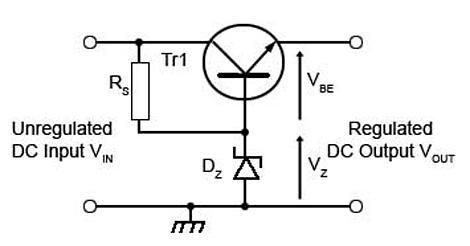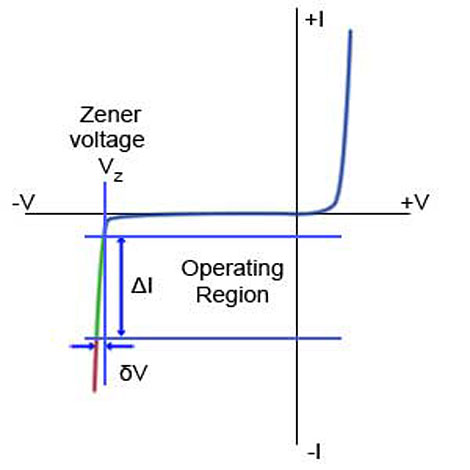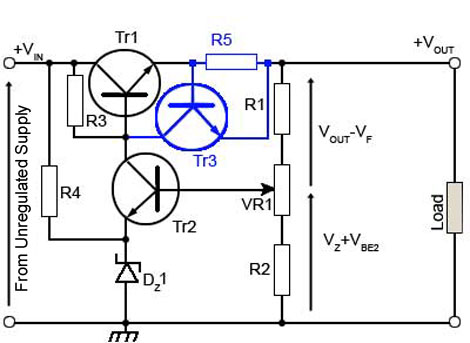# Series Voltage Regulators

• After studying this section, you should be able to:
• Understand the operation of series voltage regulators.
• • The simple series regulator.
• • Feedback and error amplification.
• • Over current protection (current limiting).
• • Over voltage protection.

## Simple Series Voltage Regulators### Fig. 2.2.1 Simple Series Regulator

In Fig. 2.2.1 RS and DZ form a simple SHUNT regulator as described in power supplies module 2.1. In this circuit however, they are used to provide a stable voltage reference VZ at the base of Tr1. The emitter voltage of Tr1 will be typically about 0.7V less than the base voltage and VOUT will therefore be at a lower voltage than the base.

VOUT = VZ - VBE

If the output voltage VOUT falls due to increased current demand by the load, this will cause VBE to increase and as a result, current through the transistor (from collector to emitter) will increase. This will provide the extra current required by the load and thus regulate the output voltage VOUT.

If VOUT tends to rise due to reduced current demand by the load, then this will reduce VBE as the emitter voltage rises and the base voltage remains stable due to DZ. This reduction in VBE will tend to turn the transistor off, reducing current flow, and again regulating the output voltage VOUT.

This regulating effect is due to the base potential of Tr1 being held steady by DZ so that any variation in emitter voltage caused by varying current flow causes a change in VBE, varying the conduction of the transistor Tr1, which will usually be a power transistor. This action counteracts the variation in load current. With this simple circuit however, regulation is not perfect, and variations in output do occur for the following reasons.### Fig. 2.2.2 Zener Diode Operating Region

1. Any increase in load current (IL) causes a small increase in base current by the ratio IL/hfe. This in turn causes an increase in VBE and because the output voltage VOUT = VZ - VBE any increase in VBE tends to reduce the output voltage. The amount of this fall is about 0.25V for a change in output current from 10mA to 1A.

2. Since base current increases with load, the current through the zener diode DZ will decrease as more current is taken by the base of Tr1. Because the diode characteristic has a slope over its operating region as shown in Fig. 2.2.2, a large change in zener current (ΔI) will cause a very small change in zener voltage (δV). This in turn will slightly affect VBE and the output voltage.

3. Because of reasons 1 and 2 above, any change in load will result in less than perfect regulation, therefore any change at the output will slightly change the loading on the input circuit. As the input is normally taken from an un-regulated supply, the input voltage will be easily affected by slight changes in load current, As the input voltage is also the supply for the reference voltage VZ any change in output current, by affecting the input voltage, can produce a noticeable effect on the output voltage, slightly reducing the effectiveness of the regulation.

Each of the above effects is small, but added together they will provide an overall effect that is noticeable when the supply is operating under demanding conditions. Nevertheless this inexpensive circuit is effective enough for many applications, and is more efficient than the shunt regulator. Also, by using a suitable power transistor, the series regulator can be used for heavier load currents than the shunt design.## Feedback and Error Amplification.

To improve on the simple series regulator a feedback circuit and error amplifier can be added to the basic series circuit.

Fig. 2.2.3 shows a block diagram of a series regulator circuit with error amplification. In this system the reference voltage VZ is compared with a feedback voltage VF, which is a portion of the actual output voltage. The difference between the two inputs produces an error voltage that is used to vary the conduction of the control element, correcting any error in the output voltage.

## Circuit Diagram.

A circuit diagram for this system is shown in Fig. 2.2.4. Tr1 is the series control element. It will usually be a power transistor, mounted on a substantial heat sink to cope with the necessary power dissipation.

A stable reference voltage is provided by R4 & D1 from the un-regulated input voltage. Tr2 is the error amplifier and its gain is set by the value of its load resistor R3. Tr2 compares the fraction of the output voltage VF fed back from the output potential divider R1/R2 with the stable reference voltage VZ across the zener diode DZ.### Fig. 2.2.4 Circuit Diagram for Fig. 2.2.3

The output voltage VOUT in Fig. 2.2.4 can be expressed as:

VOUT = (VZ + VBE2) + (VOUT - VF)

Where:

VZ is the voltage across DZ

VBE2 is the base/emitter voltage of Tr2

VF is the feedback voltage derived from the slider of VR1

Therefore:

(VZ + VBE2) is the voltage across R2 and the lower portion of VRI

and

(VOUT − VF) is the voltage across R1 and the upper portion of VRI

If the feedback voltage VF is altered by adjusting VR1 potentiometer, the difference between VF and VZ will change. This will cause a change in the error voltage controlling Tr1 and a change in the output voltage VOUT. VR1 therefore provides a variable output voltage, which, once set remains stable at that setting.

The regulating action of the circuit is governed by the voltage across the base/emitter junction of Tr2, i.e. the difference between VF and VZ.

If VOUT tends to increase, then VF - VZ also increases. This increases the collector current of Tr2 and so increases the p.d. across R3 reducing the base voltage, and therefore the base/emitter voltage of Tr1, reducing the conduction of Tr1, so reducing current flow to the load.

The output voltage VOUT is reduced in this way until a balance is reached, as the feedback portion (VF) of VOUT is also reducing. The overall effect is that the output is maintained at a level, which depends on the proportion of feedback set by the variable resistor (part of R1/R2).

If the output voltage tends to decrease, then so does VF. The base/emitter voltage of Tr2 is reduced due to the stable VZ on the emitter. Tr2 conducts less and the current through R3 falls, reducing the p.d. across it. Tr1 base voltage rises, and increases the conduction of the control transistor. This increases output current and VOUT until VF is once more at the correct level.

## Over Current Protection (Current Limiting)### Fig. 2.2.5 Series Regulator with Over Current Protection

Fig. 2.2.5 shows how the series stabiliser can be protected against excessive current being drawn by the load. This will prevent damage to the supply in the event of too much current being drawn from the output, or even a complete short circuit across the output terminals.

Two components have been added, Tr3 and R5. The resistor R5 is a very low value (typically less than 1 ohm).

When the load current rises above a predetermined value, the small voltage developed across R5 will become sufficient (at about 0.7v) to turn Tr3 on. As Tr3 is connected across the base/emitter junction of the main control transistor Tr1, the action of turning Tr3 on will reduce the base/emitter voltage of Tr1 by an amount depending on the amount of excess current. The output current will not be allowed to increase above a predetermined amount, even if a complete short circuit occurs across the output terminals. In this case Tr1 base/emitter voltage will be reduced to practically zero volts, preventing Tr1 from conducting. Under these conditions the output voltage will fall to zero for as long as the excess current condition persists, but the supply will be undamaged.## Over Voltage Protection.

Where regulated supplies are used, the DC input voltage to the regulator is often considerably higher than the required output voltage. Therefore if a PSU fault occurs, it is possible that the regulated output voltage may suddenly rise to a level that can damage other components. For this reason it is common to find over voltage protection included in stabilised supplies. The circuit shown in Fig. 2.2.6 is sometimes called a "crowbar" circuit because when it operates, it places a complete short circuit across the across the output, a similar effect to dropping a metal crowbar across the positive and ground output terminals!

## Crowbar Circuit Operation.

In Fig. 2.2.6 the zener diode DZ2 has a breakdown voltage slightly less than the maximum allowed value for VOUT. The remainder of VOUT is developed across R6, VR2 and R7.

VR2 is a potentiometer, so that a voltage may be taken from the resistor network to correctly bias the diode D1. This diode has its cathode held at 0V by R8, and VR2 is adjusted so that D1 is just out of conduction, i.e. its anode voltage is about 0.5V higher than its cathode voltage.

Now, if VOUT increases, the voltage across R6, VR2 and R7 will rise by the same amount, as the voltage across DZ2 will remain the same. Therefore there will be a substantial rise in the voltage at R7 slider, which will cause D1 to conduct, supplying a pulse of current to the gate of thyristor Th1, causing it to "fire" and conduct heavily until VOUT falls to practically 0v. R9 is included to limit the resulting current flow through the thyristor to a safe level.

The large current that flows as Th1 fires will now cause the current limiter circuit to come into operation as previously described. This will safely shut down the supply until the over current caused by Th1 has disappeared, which will of course happen as soon as VOUT reaches 0V, but should the over voltage still be present when Th1 switches off and VOUT rises again, the circuit will re-trigger, causing the voltage across the load to repeatedly alternate between its normal value and zero; a harmless but clear symptom of an over voltage problem.

Top of Page